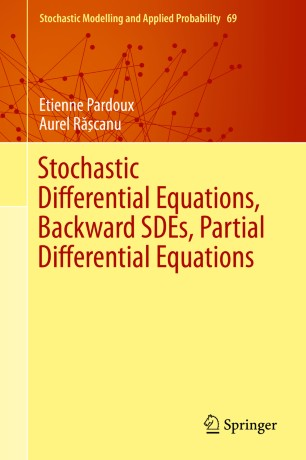# Stochastic Differential Equations, Backward SDEs, Partial Differential Equations

• Etienne Pardoux
• Aurel RӑşcanuBook

Part of the Stochastic Modelling and Applied Probability book series (SMAP, volume 69)

1. Front Matter
Pages i-xvii
2. Etienne Pardoux, Aurel Răşcanu
Pages 1-71
3. Etienne Pardoux, Aurel Răşcanu
Pages 73-133
4. Etienne Pardoux, Aurel Răşcanu
Pages 135-227
5. Etienne Pardoux, Aurel Răşcanu
Pages 229-351
6. Etienne Pardoux, Aurel Răşcanu
Pages 353-515
7. Etienne Pardoux, Aurel Răşcanu
Pages 517-658
8. Back Matter
Pages 659-667

### Introduction

This research monograph presents results to researchers in stochastic calculus, forward and backward stochastic differential equations, connections between diffusion processes and second order partial differential equations (PDEs), and financial mathematics. It pays special attention to the relations between SDEs/BSDEs and second order PDEs under minimal regularity assumptions, and also extends those results to equations with multivalued coefficients. The authors present in particular the theory of reflected SDEs in the above mentioned framework and include exercises at the end of each chapter.

Stochastic calculus and stochastic differential equations (SDEs) were first introduced by K. Itô in the 1940s, in order to construct the path of diffusion processes (which are continuous time Markov processes with continuous trajectories taking their values in a finite dimensional vector space or manifold), which had been studied from a more analytic point of view by Kolmogorov in the 1930s. Since then, this topic has become an important subject of Mathematics and Applied Mathematics, because of its mathematical richness and its importance for applications in many areas of Physics, Biology, Economics and Finance, where random processes play an increasingly important role. One important aspect is the connection between diffusion processes and linear partial differential equations of second order, which is in particular the basis for Monte Carlo numerical methods for linear PDEs. Since the pioneering work of Peng and Pardoux in the early 1990s, a new type of SDEs called backward stochastic differential equations (BSDEs) has emerged. The two main reasons why this new class of equations is important are the connection between BSDEs and semilinear PDEs, and the fact that BSDEs constitute a natural generalization of the famous Black and Scholes model from Mathematical Finance, and thus offer a natural mathematical framework for the formulation of many new models in Finance.

### Keywords

60H05, 60H10, 60J60, 35D40 Feynman-Kac formula backward stochastic differential equations reflected stochastic differential equations stochastic differential equations viscosity solutions of second-order PDEs

#### Authors and affiliations

• Etienne Pardoux
• 1
• Aurel Rӑşcanu
• 2
1. 1.Institut de Mathématiques de MarseilleUniversité d'Aix-MarseilleMarseilleFrance
2. 2.Faculty of MathematicsAlexandru Ioan Cuza UniversityIasiRomania

Industry Sectors
Pharma
Biotechnology